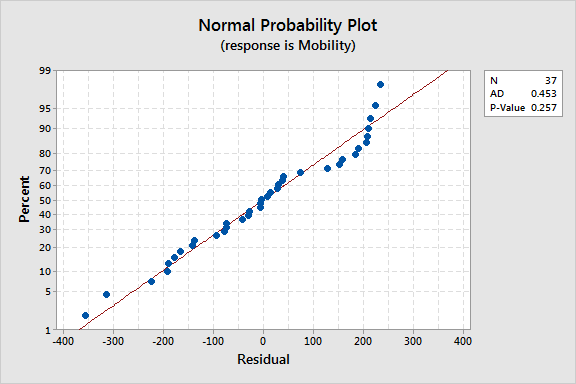Topics: Regression Analysis

I’ve written about the importance of checking your residual plots when performing linear regression analysis. If you don’t satisfy the assumptions for an analysis, you might not be able to trust the results. One of the assumptions for regression analysis is that the residuals are normally distributed. Typically, you assess this assumption using the normal probability plot of the residuals.Are these nonnormal residuals a problem?

If you have nonnormal residuals, can you trust the results of the regression analysis?

Answering this question highlights some of the research that Rob Kelly, a senior statistician here at Minitab, was tasked with in order to guide the development of our statistical software.

## Simulation Study Details

The goals of the simulation study were to:

• determine whether nonnormal residuals affect the error rate of the F-tests for regression analysis
• generate a safe, minimum sample size recommendation for nonnormal residuals

For simple regression, the study assessed both the overall F-test (for both linear and quadratic models) and the F-test specifically for the highest-order term.

For multiple regression, the study assessed the overall F-test for three models that involved five continuous predictors:

• a linear model with all five X variables
• all linear and square terms
• all linear terms and seven of the 2-way interactions

The residual distributions included skewed, heavy-tailed, and light-tailed distributions that depart substantially from the normal distribution.

There were 10,000 tests for each condition. The study determined whether the tests incorrectly rejected the null hypothesis more often or less often than expected for the different nonnormal distributions. If the test performs well, the Type I error rates should be very close to the target significance level.

## Results and Sample Size Guideline

The study found that a sample size of at least 15 was important for both simple and multiple regression. If you meet this guideline, the test results are usually reliable for any of the nonnormal distributions.

In simple regression, the observed Type I error rates are all between 0.0380 and 0.0529, very close to the target significance level of 0.05.

In multiple regression, the Type I error rates are all between 0.08820 and 0.11850, close to the target of 0.10.

## Closing Thoughts

The good news is that if you have at least 15 samples, the test results are reliable even when the residuals depart substantially from the normal distribution.

However, there is a caveat if you are using regression analysis to generate predictions. Prediction intervals are calculated based on the assumption that the residuals are normally distributed. If the residuals are nonnormal, the prediction intervals may be inaccurate.

This research guided the implementation of regression features in the Assistant menu. The Assistant is your interactive guide to choosing the right tool, analyzing data correctly, and interpreting the results. Because the regression tests perform well with relatively small samples, the Assistant does not test the residuals for normality. Instead, the Assistant checks the size of the sample and indicates when the sample is less than 15.

See a multiple regression example that uses the Assistant.

You can read the full study results in the simple regression white paper and the multiple regression white paper. You can also peruse all of our technical white papers to see the research we conduct to develop methodology throughout the Assistant and Minitab.